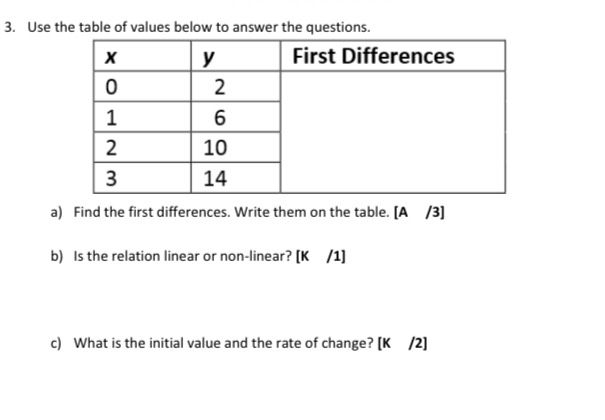### Still have math questions?

Algebra
QuestionUse the table of values below to answer the questions. Find the first differences. Write them on the table. [A $$\quad / 3 ]$$ Is the relation linear or non-linear? [ $$K \quad / 1]$$ What is the initial value and the rate of change?  $$\left[ \begin{array} { l l } { K } & { / 2 } \end{array} \right]$$

b) linear $$y = 4x + 2$$